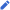# Math.NET Numerics

Developer(s) C. Rüegg, M. Cuda, et al. 4.15.0 / 7 January 2021; 5 months ago github.com/mathnet/mathnet-numericsC#, F#, .NET CLR Cross-platform Numerical library MIT/X11 numerics.mathdotnet.com

Math.NET Numerics is an open-source numerical library for .NET and Mono, written in C# and F#. It features functionality similar to BLAS and LAPACK.

## History

Math.NET Numerics started 2009 by merging code and teams of dnAnalytics with Math.NET Iridium. It is influenced by ALGLIB, JAMA and Boost, among others, and has accepted numerous code contributions. It is part of the Math.NET initiative to build and maintain open mathematical toolkits for the .NET platform since 2002.[citation needed]

Math.NET is used by several open source libraries and research projects, like MyMediaLite, FermiSim and LightField Retrieval, and various theses and papers.

## Features

The software library provides facilities for:

• Probability distributions: discrete, continuous and multivariate.
• Pseudo-random number generation, including Mersenne Twister MT19937.
• Real and complex linear algebra types and solvers with support for sparse matrices and vectors.
• LU, QR, SVD, EVD, and Cholesky decompositions.
• Matrix IO classes that read and write matrices from/to Matlab and delimited files.
• Complex number arithmetic and trigonometry.
• “Special” routines including the Gamma, Beta, Erf, modified Bessel and Struve functions.
• Interpolation routines, including Barycentric, Floater-Hormann.
• Linear Regression/Curve Fitting routines.
• Root finding methods, including Brent, Robust Newton-Raphson and Broyden.
• Descriptive Statistics, Order Statistics, Histogram, and Pearson Correlation Coefficient.
• Markov chain Monte Carlo sampling.
• Basic financial statistics.
• Fourier and Hartley transforms (FFT).
• Overloaded mathematical operators to simplify complex expressions.
• Runs under Microsoft Windows and platforms that support Mono.
• Optional support for Intel Math Kernel Library (Microsoft Windows and Linux)
• Optional F# extensions for more idiomatic usage.